RS Aggarwal (2016) Solutions for Class 10 Math Chapter 5 Trigonometric Ratios are provided here with simple step-by-step explanations. These solutions for Trigonometric Ratios are extremely popular among class 10 students for Math Trigonometric Ratios Solutions come handy for quickly completing your homework and preparing for exams. All questions and answers from the RS Aggarwal (2016) Book of class 10 Math Chapter 5 are provided here for you for free. You will also love the ad-free experience on Meritnation’s RS Aggarwal (2016) Solutions. All RS Aggarwal (2016) Solutions for class 10 Math are prepared by experts and are 100% accurate.

#### Question 1:

If sin $\mathrm{\theta }=\frac{\sqrt{3}}{2}$, find the value of all T-ratios of θ.

#### Answer:

Let us first draw a right $∆$ABC, right angled at B and $\angle C=\theta$.
Now, we know that sin $\theta$ = $\frac{\mathrm{perpendicular}}{\mathrm{hypotenuse}}$= $\frac{AB}{AC}$ = .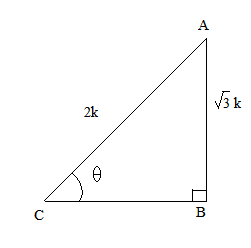So, if AB = $\sqrt{3}k$, then AC = 2k, where k is a positive number.
Now, using Pythagoras theorem, we have:
AC2 = AB2 + BC2
⇒ BC2 = AC2 $-$ AB2 = (2k)2 $-$ ($\sqrt{3}k$)2
⇒ BC2 = 4k2 $-$ 3k2 = k2
⇒ BC = k
Now, finding the other T-ratios using their definitions, we get:
cos $\theta$  = $\frac{BC}{AC}$ =
tan $\theta$  =

∴ cot $\theta$  = , cosec $\theta$ = and sec $\theta$  =

#### Question 2:

If cos  find the values of all T-ratios of θ.

#### Answer:

Let us first draw a right $∆$ABC, right angled at B and .
Now, we know that cos $\theta$ = = = .So, if BC = 7k, then AC = 25k, where k is a positive number.
Now, using Pythagoras theorem, we have:
AC2 = AB2 + BC2
⇒ AB2 = AC2 $-$ BC2 = (25k)2 $-$ (7k)2.
⇒ AB2 = 625k2 $-$ 49k2 = 576k2
⇒ AB = 24k
Now, finding the other trigonometric ratios using their definitions, we get:
sin $\theta$ = =
tan $\theta$ =
∴ cot $\theta$ = , cosec $\theta$ = and sec $\theta$  =

#### Question 3:

If tan $\mathrm{\theta }=\frac{15}{8}$ find the values of all T-ratios of θ.

#### Answer:

Let us first draw a right $∆$ABC, right angled at B and $\angle C=\theta$.
Now, we know that tan $\theta$ = $\frac{\mathrm{Perpendicular}}{\mathrm{Base}}$ = $\frac{AB}{BC}$ = $\frac{15}{8}$.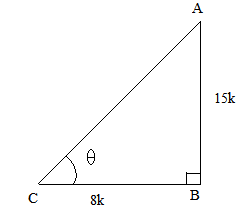So, if BC = 8k, then AB = 15k, where k is a positive number.
Now, using Pythagoras theorem, we have:
AC2 = AB2 + BC2 = (15k)2 + (8k)2
⇒ AC2 = 225k2 + 64k2 = 289k2
⇒ AC = 17k

Now, finding the other T-ratios using their definitions, we get:
sin $\theta$  = $\frac{AB}{AC}$ =
cos $\theta$  =

∴ cot $\theta$  = , cosec $\theta$ = and sec $\theta$  =

#### Question 4:

If cot θ = 2, find the value of all T-ratios of θ.

#### Answer:

Let us first draw a right $∆$ABC, right angled at B and $\angle C=\theta$.
Now, we know that cot $\theta$$\frac{\mathrm{base}}{\mathrm{Perpendicular}}$ = $\frac{BC}{AB}$ = 2.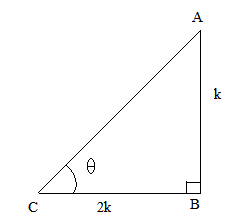So, if BC = 2k, then AB = k, where k is a positive number.
Now, using Pythagoras theorem, we have:
AC2 = AB2 + BC2 = (2k)2 + (k)2
⇒ AC2 = 4k2 + k2 = 5k2
⇒ AC = $\sqrt{5}$k
Now, finding the other T-ratios using their definitions, we get:
sin $\theta$  = $\frac{AB}{AC}$ =
cos $\theta$  =

∴ tan $\theta$  = , cosec $\theta$ = and sec $\theta$  =

#### Question 5:

If cosec θ = $\sqrt{10}$, the find the values of all T-ratios of θ.

#### Answer:

Let us first draw a right $∆$ABC, right angled at B and $\angle C=\theta$.
Now, we know that cosec $\theta$ = $\frac{\mathrm{Hypotenuse}}{\mathrm{Perpendicular}}$ = $\frac{AC}{AB}$= $\frac{\sqrt{10}}{1}$.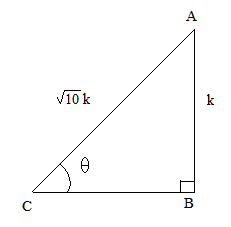So, if AC = ($\sqrt{10}$)k, then AB = k, where k is a positive number.
Now, by using Pythagoras theorem, we have:
AC2 = AB2 + BC2
⇒ BC2 = AC2 $-$ AB2 = 10k2 $-$ k2
⇒ BC2 = 9k2
⇒ BC = 3k
Now, finding the other T-ratios using their definitions, we get:
tan $\theta$  = $\frac{AB}{BC}$ =

cos $\theta$  =

∴ , cot $\theta$  = and sec $\theta$  =

#### Question 6:

If $\mathrm{sin}\theta =\frac{{a}^{2}-{b}^{2}}{{a}^{2}+{b}^{2}}$, find the values of all T-ratios of $\theta$.

#### Answer:

We have $\mathrm{sin}\theta =\frac{{a}^{2}-{b}^{2}}{{a}^{2}+{b}^{2}}$,

As,

${\mathrm{cos}}^{2}\theta =1-{\mathrm{sin}}^{2}\theta \phantom{\rule{0ex}{0ex}}=1-{\left(\frac{{a}^{2}-{b}^{2}}{{a}^{2}+{b}^{2}}\right)}^{2}\phantom{\rule{0ex}{0ex}}=\frac{1}{1}-\frac{{\left({a}^{2}-{b}^{2}\right)}^{2}}{{\left({a}^{2}+{b}^{2}\right)}^{2}}\phantom{\rule{0ex}{0ex}}=\frac{{\left({a}^{2}+{b}^{2}\right)}^{2}-{\left({a}^{2}-{b}^{2}\right)}^{2}}{{\left({a}^{2}+{b}^{2}\right)}^{2}}\phantom{\rule{0ex}{0ex}}=\frac{\left[\left({a}^{2}+{b}^{2}\right)-\left({a}^{2}-{b}^{2}\right)\right]\left[\left({a}^{2}+{b}^{2}\right)+\left({a}^{2}-{b}^{2}\right)\right]}{{\left({a}^{2}+{b}^{2}\right)}^{2}}$
$=\frac{\left[{a}^{2}+{b}^{2}-{a}^{2}+{b}^{2}\right]\left[{a}^{2}+{b}^{2}+{a}^{2}-{b}^{2}\right]}{{\left({a}^{2}+{b}^{2}\right)}^{2}}\phantom{\rule{0ex}{0ex}}=\frac{\left[2{b}^{2}\right]\left[2{a}^{2}\right]}{{\left({a}^{2}+{b}^{2}\right)}^{2}}\phantom{\rule{0ex}{0ex}}⇒{\mathrm{cos}}^{2}\theta =\frac{4{a}^{2}{b}^{2}}{{\left({a}^{2}+{b}^{2}\right)}^{2}}\phantom{\rule{0ex}{0ex}}⇒\mathrm{cos}\theta =\sqrt{\frac{4{a}^{2}{b}^{2}}{{\left({a}^{2}+{b}^{2}\right)}^{2}}}\phantom{\rule{0ex}{0ex}}⇒\mathrm{cos}\theta =\frac{2ab}{\left({a}^{2}+{b}^{2}\right)}$

Also,

$\mathrm{tan}\theta =\frac{\mathrm{sin}\theta }{\mathrm{cos}\theta }\phantom{\rule{0ex}{0ex}}=\frac{\left(\frac{{a}^{2}-{b}^{2}}{{a}^{2}+{b}^{2}}\right)}{\left(\frac{2ab}{{a}^{2}+{b}^{2}}\right)}\phantom{\rule{0ex}{0ex}}=\frac{{a}^{2}-{b}^{2}}{2ab}$

Now,

$\mathrm{cosec}\theta =\frac{1}{\mathrm{sin}\theta }\phantom{\rule{0ex}{0ex}}=\frac{1}{\left(\frac{{a}^{2}-{b}^{2}}{{a}^{2}+{b}^{2}}\right)}\phantom{\rule{0ex}{0ex}}=\frac{{a}^{2}+{b}^{2}}{{a}^{2}-{b}^{2}}$

Also,

$\mathrm{sec}\theta =\frac{1}{\mathrm{cos}\theta }\phantom{\rule{0ex}{0ex}}=\frac{1}{\left(\frac{2ab}{{a}^{2}+{b}^{2}}\right)}\phantom{\rule{0ex}{0ex}}=\frac{{a}^{2}+{b}^{2}}{2ab}$

And,

$\mathrm{cot}\theta =\frac{1}{\mathrm{tan}\theta }\phantom{\rule{0ex}{0ex}}=\frac{1}{\left(\frac{{a}^{2}-{b}^{2}}{2ab}\right)}\phantom{\rule{0ex}{0ex}}=\frac{2ab}{{a}^{2}-{b}^{2}}$

#### Question 7:

If $15\mathrm{cot}A=8$, find the values of sinA and secA.

#### Answer:

We have,

$15\mathrm{cot}A=8\phantom{\rule{0ex}{0ex}}⇒\mathrm{cot}A=\frac{8}{15}$

As,

${\mathrm{cosec}}^{2}A=1+{\mathrm{cot}}^{2}A\phantom{\rule{0ex}{0ex}}=1+{\left(\frac{8}{15}\right)}^{2}\phantom{\rule{0ex}{0ex}}=1+\frac{64}{225}\phantom{\rule{0ex}{0ex}}=\frac{225+64}{225}$
$⇒{\mathrm{cosec}}^{2}A=\frac{289}{225}\phantom{\rule{0ex}{0ex}}⇒\mathrm{cosec}A=\sqrt{\frac{289}{225}}\phantom{\rule{0ex}{0ex}}⇒\mathrm{cosec}A=\frac{17}{15}\phantom{\rule{0ex}{0ex}}⇒\frac{1}{\mathrm{sin}A}=\frac{17}{15}\phantom{\rule{0ex}{0ex}}⇒\mathrm{sin}A=\frac{15}{17}$

Also,

${\mathrm{cos}}^{2}A=1-{\mathrm{sin}}^{2}A\phantom{\rule{0ex}{0ex}}=1-{\left(\frac{15}{17}\right)}^{2}\phantom{\rule{0ex}{0ex}}=1-\frac{225}{289}\phantom{\rule{0ex}{0ex}}=\frac{289-225}{289}$
$⇒{\mathrm{cos}}^{2}A=\frac{64}{289}\phantom{\rule{0ex}{0ex}}⇒\mathrm{cos}A=\sqrt{\frac{64}{289}}\phantom{\rule{0ex}{0ex}}⇒\mathrm{cos}A=\frac{8}{17}\phantom{\rule{0ex}{0ex}}⇒\frac{1}{\mathrm{sec}A}=\frac{8}{17}\phantom{\rule{0ex}{0ex}}⇒\mathrm{sec}A=\frac{17}{8}$

#### Question 8:

If $\mathrm{sin}A=\frac{9}{41}$, find the values of cosA and tanA.

#### Answer:

We have $\mathrm{sin}A=\frac{9}{41}$,

As,

${\mathrm{cos}}^{2}A=1-{\mathrm{sin}}^{2}A\phantom{\rule{0ex}{0ex}}=1-{\left(\frac{9}{41}\right)}^{2}\phantom{\rule{0ex}{0ex}}=1-\frac{81}{1681}\phantom{\rule{0ex}{0ex}}=\frac{1681-81}{1681}$
$⇒{\mathrm{cos}}^{2}\mathrm{A}=\frac{1600}{1681}\phantom{\rule{0ex}{0ex}}⇒\mathrm{cos}A=\sqrt{\frac{1600}{1681}}\phantom{\rule{0ex}{0ex}}⇒\mathrm{cos}A=\frac{40}{41}$

Also,

$\mathrm{tan}A=\frac{\mathrm{sin}A}{\mathrm{cos}A}\phantom{\rule{0ex}{0ex}}=\frac{\left(\frac{9}{41}\right)}{\left(\frac{40}{41}\right)}\phantom{\rule{0ex}{0ex}}=\frac{9}{40}$

#### Question 9:

If cos θ = 0.6, show that (5 sin θ − 3 tan θ) = 0.

#### Answer:

Let us consider a right $△$ABC right angled at B.
Now, we know that cos $\theta$ = 0.6 = $\frac{BC}{AC}$$\frac{3}{5}$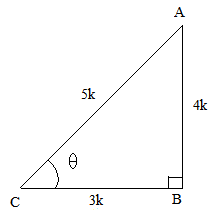So, if BC = 3k, then AC = 5k, where k is a positive number.
Using Pythagoras theorem, we have:
AC2 = AB2  + BC2
⇒ AB2 = AC2 $-$ BC2
⇒ AB2 = (5k)2 $-$ (3k)2 = 25k2 $-$ 9k2
⇒ AB2 = 16k2
⇒ AB = 4k

Finding out the other T-ratios using their definitions, we get:
sin $\theta$ =

tan $\theta$ =

Substituting the values in the given expression, we get:
5 sin $\theta$ $-$ 3 tan $\theta$

i.e., LHS  = RHS

Hence proved.

#### Question 10:

If cosec θ = 2, show that $\left(\mathrm{cot\theta }+\frac{\mathrm{sin\theta }}{1+\mathrm{cos\theta }}\right)=2.$

#### Answer:

Let us consider a right $△$ABC, right angled at B and $\angle C=\theta$.
Now, it is given that cosec $\theta$ = 2.
Also, sin $\theta$  =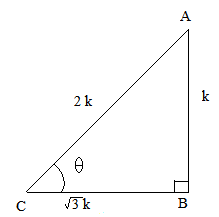So, if AB = k, then AC = 2k, where k is a positive number.
Using Pythagoras theorem, we have:
⇒ AC2 = AB2  + BC2
⇒ BC2 = AC2 $-$ AB2
⇒ BC2 = (2k)2 $-$ (k)2
⇒ BC2 = 3k2
⇒ BC = $\sqrt{3}$k
Finding out the other T-ratios using their definitions, we get:
cos $\theta$ =
tan $\theta$ =
cot $\theta$ =
Substituting these values in the given expression, we get:

$=\sqrt{3}+\frac{1}{2+\sqrt{3}}\phantom{\rule{0ex}{0ex}}=\frac{\sqrt{3}\left(2+\sqrt{3}\right)+1}{2+\sqrt{3}}\phantom{\rule{0ex}{0ex}}=\frac{2\sqrt{3}+3+1}{2+\sqrt{3}}\phantom{\rule{0ex}{0ex}}=\frac{2\left(2+\sqrt{3}\right)}{2+\sqrt{3}}=2$
i.e., LHS = RHS

Hence proved.

#### Question 11:

If tan θ = $\frac{1}{\sqrt{7}}$, show that $\frac{\left({\mathrm{cosec}}^{2}\mathrm{\theta }-{\mathrm{sec}}^{2}\mathrm{\theta }\right)}{\left({\mathrm{cosec}}^{2}\mathrm{\theta }+{\mathrm{sec}}^{2}\mathrm{\theta }\right)}=\frac{3}{4}.$

#### Answer:

Let us consider a right $△$ABC, right angled at B and $\angle C=\theta$.
Now it is given that tan $\theta$$\frac{AB}{BC}$$\frac{1}{\sqrt{7}}$.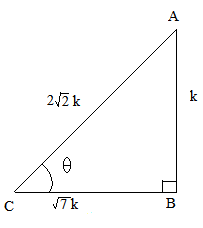So, if AB = k, then BC = $\sqrt{7}$k, where k is a positive number.
Using Pythagoras theorem, we have:
AC2 = AB2 + BC2
⇒ AC2 = (k)2 + ($\sqrt{7}$k)2
⇒ AC2 = k2 + 7k2
⇒ AC = 2$\sqrt{2}$k
Now, finding out the values of the other trigonometric ratios, we have:
sin $\theta$  =
cos $\theta$  =
∴ cosec $\theta$  = and sec $\theta$   =
Substituting the values of cosec $\theta$  and sec $\theta$  in the given expression, we get:

i.e., LHS = RHS

Hence proved.

#### Question 12:

If tan θ = $\frac{20}{21}$, show that$\frac{\left(1-\mathrm{sin\theta }+\mathrm{cos\theta }\right)}{\left(1+\mathrm{sin\theta }+\mathrm{cos\theta }\right)}=\frac{3}{7}.$

#### Answer:

Let us consider a right $△$ABC right angled at B and $\angle C=\theta$.
Now, we know that tan $\theta$$\frac{AB}{BC}$ = $\frac{20}{21}$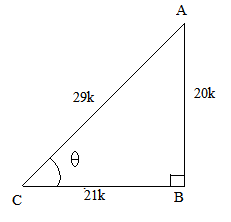So, if AB = 20k, then BC = 21k, where k is a positive number.
Using Pythagoras theorem, we get:
AC2 = AB2 + BC2
⇒ AC2= (20k)2 + (21k)2
⇒ AC2 = 841k2
⇒  AC = 29k
Now, sin $\theta$ = and cos $\theta$ =

Substituting these values in the given expression, we get:

∴ LHS = RHS

Hence proved.

#### Question 13:

If $\mathrm{sec}\theta =\frac{5}{4}$, show that $\frac{\left(\mathrm{sin}\theta -2\mathrm{cos}\theta \right)}{\left(\mathrm{tan}\theta -\mathrm{cot}\theta \right)}=\frac{12}{7}$.

#### Answer:

We have,

$\mathrm{sec}\theta =\frac{5}{4}\phantom{\rule{0ex}{0ex}}⇒\frac{1}{\mathrm{cos}\theta }=\frac{5}{4}\phantom{\rule{0ex}{0ex}}⇒\mathrm{cos}\theta =\frac{4}{5}$

Also,

${\mathrm{sin}}^{2}\theta =1-{\mathrm{cos}}^{2}\theta \phantom{\rule{0ex}{0ex}}=1-{\left(\frac{4}{5}\right)}^{2}\phantom{\rule{0ex}{0ex}}=1-\frac{16}{25}\phantom{\rule{0ex}{0ex}}=\frac{9}{25}\phantom{\rule{0ex}{0ex}}⇒\mathrm{sin}\theta =\frac{3}{5}$

Now,

$=\frac{\frac{3}{5}×\frac{4}{5}\left(\frac{3}{5}-2×\frac{4}{5}\right)}{{\left(\frac{3}{5}\right)}^{2}-{\left(\frac{4}{5}\right)}^{2}}\phantom{\rule{0ex}{0ex}}=\frac{\frac{12}{25}\left(\frac{3}{5}-\frac{8}{5}\right)}{\left(\frac{9}{25}-\frac{16}{25}\right)}\phantom{\rule{0ex}{0ex}}=\frac{\frac{12}{25}×\left(\frac{-5}{5}\right)}{\left(\frac{-7}{25}\right)}\phantom{\rule{0ex}{0ex}}=\frac{12}{7}\phantom{\rule{0ex}{0ex}}=\mathrm{RHS}$

#### Question 14:

If $\mathrm{cot}\theta =\frac{3}{4}$, show that $\sqrt{\frac{\mathrm{sec}\theta -\mathrm{cosec}\theta }{\mathrm{sec}\theta +\mathrm{cosec}\theta }}=\frac{1}{\sqrt{7}}$.

#### Answer:

$=\sqrt{\frac{\left(\frac{1}{4}\right)}{\left(\frac{7}{4}\right)}}\phantom{\rule{0ex}{0ex}}=\sqrt{\frac{1}{7}}\phantom{\rule{0ex}{0ex}}=\frac{1}{\sqrt{7}}\phantom{\rule{0ex}{0ex}}=\mathrm{RHS}$

#### Question 15:

If $\mathrm{sin}\theta =\frac{3}{4}$, show that $\sqrt{\frac{{\mathrm{cosec}}^{2}\theta -{\mathrm{cot}}^{2}\theta }{{\mathrm{sec}}^{2}\theta -1}}=\frac{\sqrt{7}}{3}$.

#### Answer:

$\mathrm{LHS}=\sqrt{\frac{{\mathrm{cosec}}^{2}\theta -{\mathrm{cot}}^{2}\theta }{{\mathrm{sec}}^{2}\theta -1}}\phantom{\rule{0ex}{0ex}}=\sqrt{\frac{1}{{\mathrm{tan}}^{2}\theta }}\phantom{\rule{0ex}{0ex}}=\sqrt{{\mathrm{cot}}^{2}\theta }\phantom{\rule{0ex}{0ex}}=\mathrm{cot}\theta \phantom{\rule{0ex}{0ex}}=\sqrt{{\mathrm{cosec}}^{2}\theta -1}\phantom{\rule{0ex}{0ex}}=\sqrt{{\left(\frac{1}{\mathrm{sin}\theta }\right)}^{2}-1}\phantom{\rule{0ex}{0ex}}=\sqrt{{\left(\frac{1}{\left(\frac{3}{4}\right)}\right)}^{2}-1}\phantom{\rule{0ex}{0ex}}=\sqrt{{\left(\frac{4}{3}\right)}^{2}-1}\phantom{\rule{0ex}{0ex}}=\sqrt{\frac{16}{9}-1}\phantom{\rule{0ex}{0ex}}=\sqrt{\frac{16-9}{9}}\phantom{\rule{0ex}{0ex}}=\sqrt{\frac{7}{9}}\phantom{\rule{0ex}{0ex}}=\frac{\sqrt{7}}{3}\phantom{\rule{0ex}{0ex}}=\mathrm{RHS}$

#### Question 16:

If $\mathrm{sin}\theta =\frac{a}{b}$, show that $\left(\mathrm{sec}\theta +\mathrm{tan}\theta \right)=\sqrt{\frac{b+a}{b-a}}$.

#### Answer:

$\mathrm{LHS}=\left(\mathrm{sec}\theta +\mathrm{tan}\theta \right)\phantom{\rule{0ex}{0ex}}=\frac{1}{\mathrm{cos}\theta }+\frac{\mathrm{sin}\theta }{\mathrm{cos}\theta }\phantom{\rule{0ex}{0ex}}=\frac{1+\mathrm{sin}\theta }{\mathrm{cos}\theta }\phantom{\rule{0ex}{0ex}}=\frac{1+\mathrm{sin}\theta }{\sqrt{1-{\mathrm{sin}}^{2}\theta }}\phantom{\rule{0ex}{0ex}}=\frac{\left(1+\frac{a}{b}\right)}{\sqrt{1-{\left(\frac{a}{b}\right)}^{2}}}$
$=\frac{\left(\frac{1}{1}+\frac{a}{b}\right)}{\sqrt{\frac{1}{1}-\frac{{a}^{2}}{{b}^{2}}}}\phantom{\rule{0ex}{0ex}}=\frac{\left(\frac{b+a}{b}\right)}{\sqrt{\frac{{b}^{2}-{a}^{2}}{{b}^{2}}}}\phantom{\rule{0ex}{0ex}}=\frac{\left(\frac{b+a}{b}\right)}{\left(\frac{\sqrt{{b}^{2}-{a}^{2}}}{b}\right)}\phantom{\rule{0ex}{0ex}}=\frac{\left(b+a\right)}{\sqrt{\left(b+a\right)\left(b-a\right)}}$
$=\frac{\left(b+a\right)}{\sqrt{\left(b+a\right)}\sqrt{\left(b-a\right)}}\phantom{\rule{0ex}{0ex}}=\frac{\sqrt{\left(b+a\right)}}{\sqrt{\left(b-a\right)}}\phantom{\rule{0ex}{0ex}}=\sqrt{\frac{b+a}{b-a}}\phantom{\rule{0ex}{0ex}}=\mathrm{RHS}$

#### Question 17:

If $\mathrm{cos}\theta =\frac{3}{5}$, show that $\frac{\left(\mathrm{sin}\theta -\mathrm{cot}\theta \right)}{2\mathrm{tan}\theta }=\frac{3}{160}$.

#### Answer:

$\mathrm{LHS}=\frac{\left(\mathrm{sin}\theta -\mathrm{cot}\theta \right)}{2\mathrm{tan}\theta }\phantom{\rule{0ex}{0ex}}=\frac{\left(\mathrm{sin}\theta -\frac{\mathrm{cos}\theta }{\mathrm{sin}\theta }\right)}{2\left(\frac{\mathrm{sin}\theta }{\mathrm{cos}\theta }\right)}\phantom{\rule{0ex}{0ex}}=\frac{\left(\frac{{\mathrm{sin}}^{2}\theta -\mathrm{cos}\theta }{\mathrm{sin}\theta }\right)}{\left(\frac{2\mathrm{sin}\theta }{\mathrm{cos}\theta }\right)}\phantom{\rule{0ex}{0ex}}=\frac{\mathrm{cos}\theta \left({\mathrm{sin}}^{2}\theta -\mathrm{cos}\theta \right)}{2{\mathrm{sin}}^{2}\theta }\phantom{\rule{0ex}{0ex}}=\frac{\mathrm{cos}\theta \left(1-{\mathrm{cos}}^{2}\theta -\mathrm{cos}\theta \right)}{2\left(1-{\mathrm{cos}}^{2}\theta \right)}$
$=\frac{\frac{3}{5}\left[1-{\left(\frac{3}{5}\right)}^{2}-\frac{3}{5}\right]}{2\left[1-{\left(\frac{3}{5}\right)}^{2}\right]}\phantom{\rule{0ex}{0ex}}=\frac{\frac{3}{5}\left(\frac{1}{1}-\frac{9}{25}-\frac{3}{5}\right)}{2\left(1-\frac{9}{25}\right)}\phantom{\rule{0ex}{0ex}}=\frac{\frac{3}{5}\left(\frac{25-9-15}{25}\right)}{2\left(\frac{25-9}{25}\right)}\phantom{\rule{0ex}{0ex}}=\frac{\frac{3}{5}\left(\frac{1}{25}\right)}{2\left(\frac{16}{25}\right)}$
$=\frac{3}{5×2×16}\phantom{\rule{0ex}{0ex}}=\frac{3}{160}\phantom{\rule{0ex}{0ex}}=\mathrm{RHS}$

#### Question 18:

If tan θ = $\frac{4}{3}$, show that (sin θ + cos θ) = $\frac{7}{5}$.

#### Answer:

Let us consider a right $△$ABC, right angled at B and $\angle C=\theta$.
Now, we know that  tan $\theta$$\frac{AB}{BC}$$\frac{4}{3}$.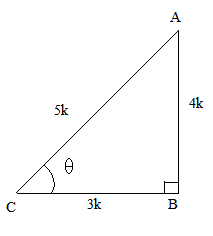So, if BC = 3k, then AB = 4k, where k is a positive number.
Using Pythagoras theorem, we have:
AC2 = AB2 + BC2 = (4k)2 + (3k)2
⇒ AC2 = 16k2 + 9k2 = 25k2
⇒ AC = 5k
Finding out the values of sin $\theta$ and cos $\theta$ using their definitions, we have:
sin $\theta$ =
cos $\theta$ =
Substituting these values in the given expression, we get:
(sin $\theta$ + cos $\theta$) =
i.e., LHS = RHS

Hence proved.

#### Question 19:

If tan θ = $\frac{a}{b}$, show that

#### Answer:

It is given that tan .

LHS =
Dividing the numerator and denominator by cos $\theta$, we get:

(∵ tan )
Now, substituting the value of tan $\theta$ in the above expression, we get:

i.e., LHS = RHS

Hence proved.

#### Question 20:

If 3 tan θ = 4, show that $\frac{4\mathrm{cos\theta }-\mathrm{sin\theta }}{2\mathrm{cos\theta }+\mathrm{sin\theta }}=\frac{4}{5}.$

#### Answer:

Let us consider a right $△$ABC right angled at B and $\angle C=\theta$.
We know that tan $\theta$ = $\frac{AB}{BC}$$\frac{4}{3}$So, if BC = 3k, then AB = 4k, where k is a positive number.
Using Pythagoras theorem, we have:
AC2 = AB2  + BC2
⇒ AC2 = 16k2 + 9k2
⇒ AC2 = 25k2
⇒ AC = 5k

Now, we have:

sin $\theta$ =

cos $\theta$ =

Substituting these values in the given expression, we get:

i.e., LHS = RHS

Hence proved.

#### Question 21:

If 3 cot θ = 2, show that $\left(\frac{4\mathrm{sin\theta }-3\mathrm{cos\theta }}{2\mathrm{sin\theta }+6\mathrm{cos\theta }}\right)=\frac{1}{3}.$

#### Answer:

It is given that cot .

LHS  =
Dividing the above expression by sin $\theta$, we get:
[∵ cot ]
Now, substituting the values of cot $\theta$ in the above expression, we get:

i.e., LHS = RHS

Hence proved.

#### Question 22:

If $3\mathrm{cot}\theta =4$, show that $\frac{\left(1-{\mathrm{tan}}^{2}\theta \right)}{\left(1+{\mathrm{tan}}^{2}\theta \right)}=\left({\mathrm{cos}}^{2}\theta -{\mathrm{sin}}^{2}\theta \right)$.

#### Answer:

$=\frac{\frac{16}{9}-1}{\frac{16}{9}+1}\phantom{\rule{0ex}{0ex}}=\frac{\left(\frac{16-9}{9}\right)}{\left(\frac{16+9}{9}\right)}\phantom{\rule{0ex}{0ex}}=\frac{\left(\frac{7}{9}\right)}{\left(\frac{25}{9}\right)}\phantom{\rule{0ex}{0ex}}=\frac{7}{25}$
$\mathrm{RHS}=\left({\mathrm{cos}}^{2}\theta -{\mathrm{sin}}^{2}\theta \right)\phantom{\rule{0ex}{0ex}}=\frac{\left({\mathrm{cos}}^{2}\theta -{\mathrm{sin}}^{2}\theta \right)}{1}\phantom{\rule{0ex}{0ex}}=\frac{\left(\frac{{\mathrm{cos}}^{2}\theta -{\mathrm{sin}}^{2}\theta }{{\mathrm{sin}}^{2}\theta }\right)}{\left(\frac{1}{{\mathrm{sin}}^{2}\theta }\right)}\phantom{\rule{0ex}{0ex}}=\frac{\left(\frac{{\mathrm{cos}}^{2}\theta }{{\mathrm{sin}}^{2}\theta }-\frac{{\mathrm{sin}}^{2}\theta }{{\mathrm{sin}}^{2}\theta }\right)}{{\mathrm{cosec}}^{2}\theta }\phantom{\rule{0ex}{0ex}}=\frac{\left({\mathrm{cot}}^{2}\theta -1\right)}{\left({\mathrm{cot}}^{2}\theta +1\right)}$
$=\frac{\left[{\left(\frac{4}{3}\right)}^{2}-1\right]}{\left[{\left(\frac{4}{3}\right)}^{2}+1\right]}\phantom{\rule{0ex}{0ex}}=\frac{\left(\frac{16}{9}-\frac{1}{1}\right)}{\left(\frac{16}{9}+\frac{1}{1}\right)}\phantom{\rule{0ex}{0ex}}=\frac{\left(\frac{16-9}{9}\right)}{\left(\frac{16+9}{9}\right)}\phantom{\rule{0ex}{0ex}}=\frac{\left(\frac{7}{9}\right)}{\left(\frac{25}{9}\right)}\phantom{\rule{0ex}{0ex}}=\frac{7}{25}$

Since, LHS=RHS
Hence, verified.

#### Question 23:

If sec θ = $\frac{17}{8}$, verify that $\frac{3-4{\mathrm{sin}}^{2}\mathrm{\theta }}{4{\mathrm{cos}}^{2}\mathrm{\theta }-3}=\frac{3-{\mathrm{tan}}^{2}\mathrm{\theta }}{1-3{\mathrm{tan}}^{2}\mathrm{\theta }}$.

#### Answer:

It is given that sec $\theta$ = $\frac{17}{8}$.

Let us consider a right $△$ABC right angled at B and $\angle C=\theta$.
We know that cos $\theta$ =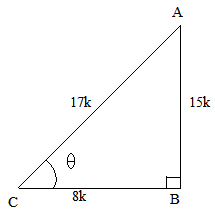So, if BC = 8k, then AC = 17k, where k is a positive number.
Using Pythagoras theorem, we have:
AC2 = AB2 + BC2
⇒ AB2 = AC2 $-$ BC2 = (17k)2 $-$ (8k)2
⇒ AB2 = 289k2 $-$ 64k2 = 225k2
⇒ AB = 15k.

Now, tan $\theta$  = and sin $\theta$ =

The given expression is .

Substituting the values in the above expression, we get:

∴ LHS = RHS
Hence proved.

#### Question 24:

In the adjoining figure,#### Answer:In $∆$ABD,

Using Pythagoras theorem, we get

Again,

In $∆$ABC,

Using Pythagoras therem, we get

Now,

#### Question 25:

In a ∆ABC, it is given that ∠B = 90°, AB = 24 cm and BC = 7 cm.
Figure

Find the value of
(i) sin A
(ii) cos A
(iii) sin C
(iv) cos C

#### Answer: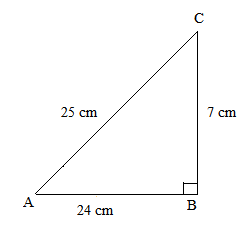Using Pythagoras theorem, we get:
AC2 = AB2 + BC2
⇒ AC2= (24)2 + (7)2
⇒ AC2 = 576 + 49 = 625
⇒ AC = 25 cm
Now, for T-Ratios of ∠A, base = AB and perpendicular = BC
(i)  sin A =

(ii) cos A =

Similarly, for T-Ratios of ∠C, base = BC and perpendicular = AB
(iii) sin C =

(iv) cos C =

#### Question 26:

Given a ∆ABC, in which ∠C = 90°, ∠ABC = θ°, BC = 21 units, AB = 29 units.
Show that (cos2θ − sin2θ) = $\frac{41}{841}.$

#### Answer: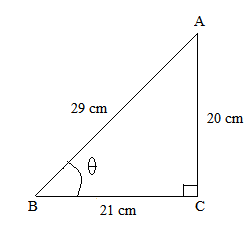Using Pythagoras theorem, we get:
AB2  =  AC2  + BC2
⇒ AC2 = AB2 $-$ BC2
⇒ AC2 = (29)2 $-$ (21)2
⇒ AC2 = 841$-$ 441
⇒ AC2 = 400
⇒ AC = $\sqrt{400}$ = 20 units

Now, sin and cos $\theta$ =

cos2 $\theta$ $-$ sin2 $\theta$ =

Hence Proved.

#### Question 27:

In a ∆ABC, right-angled at B, it is given that AB = 12 cm and BC = 5 cm.

Find the value of
(i) cos A
(ii) cosec A
(iii) cos C
(iv) coses C.

#### Answer: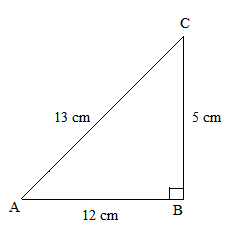Using Pythagoras theorem, we get:
AC2 = AB2 + BC2
⇒ AC2 = 122  + 52 = 144 + 25
⇒ AC2 = 169
⇒ AC = 13 cm
Now, for T-Ratios of ∠A, base = AB and perpendicular = BC
(i) cos A =
(ii) cosec A =

Similarly, for T-Ratios of ∠C, base = BC and perpendicular = AB
(iii) cos C =
(iv) cosec C =

#### Question 28:

If $\mathrm{sin}\alpha =\frac{1}{2}$, prove that $\left(3\mathrm{cos}\alpha -4{\mathrm{cos}}^{3}\alpha \right)=0$.

#### Answer:

$\mathrm{LHS}=\left(3\mathrm{cos}\alpha -4{\mathrm{cos}}^{3}\alpha \right)\phantom{\rule{0ex}{0ex}}=\mathrm{cos}\alpha \left(3-4{\mathrm{cos}}^{2}\alpha \right)\phantom{\rule{0ex}{0ex}}=\sqrt{1-{\mathrm{sin}}^{2}\alpha }\left[3-4\left(1-{\mathrm{sin}}^{2}\alpha \right)\right]\phantom{\rule{0ex}{0ex}}=\sqrt{1-{\left(\frac{1}{2}\right)}^{2}}\left[3-4\left(1-{\left(\frac{1}{2}\right)}^{2}\right)\right]\phantom{\rule{0ex}{0ex}}=\sqrt{\frac{1}{1}-\frac{1}{4}}\left[3-4\left(\frac{1}{1}-\frac{1}{4}\right)\right]\phantom{\rule{0ex}{0ex}}=\sqrt{\frac{3}{4}}\left[3-4\left(\frac{3}{4}\right)\right]\phantom{\rule{0ex}{0ex}}=\sqrt{\frac{3}{4}}\left[3-3\right]\phantom{\rule{0ex}{0ex}}=\sqrt{\frac{3}{4}}\left[0\right]\phantom{\rule{0ex}{0ex}}=0\phantom{\rule{0ex}{0ex}}=\mathrm{RHS}$

#### Question 29:

In a $∆$ABC, $\angle$B = 90$°$ and tanA = $\frac{1}{\sqrt{3}}$. Prove that
(i) sinA$·$cosC + cosA$·$sinC = 1            (ii) cosA$·$cosC $-$ sinA$·$sinC = 0

#### Answer:Now,

#### Question 30:

If $\angle$A and $\angle$B are acute angles such that sinA = sinB, then prove that $\angle$A = $\angle$B.

#### Answer:In $∆$ABC, $\angle$C = 90$°$
sinA = $\frac{\mathrm{BC}}{\mathrm{AB}}$ and
sinB = $\frac{\mathrm{AC}}{\mathrm{AB}}$

As, sinA = sinB
$⇒$$\frac{\mathrm{BC}}{\mathrm{AB}}$ = $\frac{\mathrm{AC}}{\mathrm{AB}}$
$⇒$BC = AC
So, $\angle$A = $\angle$B             (Angles opposite to equal sides are equal)

#### Question 31:

If $\angle \mathrm{A}$ and $\angle \mathrm{B}$ are acute angles such that tanA = tanB, the prove that $\angle \mathrm{A}=\angle \mathrm{B}$.

#### Answer:In ,

#### Question 32:

In a right $∆\mathrm{ABC}$, right-angled at $\mathrm{B}$, if $\mathrm{tanA}=1$, then verify that $2\mathrm{sinA}·\mathrm{cosA}=1$.

#### Question 33:

In the figure of . Find#### Answer:In ,

Using Pythagoras theorem, we get

$\mathrm{PQ}=\sqrt{{\mathrm{PR}}^{2}-{\mathrm{QR}}^{2}}\phantom{\rule{0ex}{0ex}}=\sqrt{{\left(x+2\right)}^{2}-{x}^{2}}\phantom{\rule{0ex}{0ex}}=\sqrt{{x}^{2}+4x+4-{x}^{2}}\phantom{\rule{0ex}{0ex}}=\sqrt{4\left(x+1\right)}\phantom{\rule{0ex}{0ex}}=2\sqrt{x+1}$

Now,

#### Question 34:

If $x=\mathrm{cosecA}+\mathrm{cosA}$ and $y=\mathrm{cosecA}-\mathrm{cosA}$, then prove that ${\left(\frac{2}{x+y}\right)}^{2}+{\left(\frac{x-y}{2}\right)}^{2}-1=0$.

#### Answer:

$\mathrm{LHS}={\left(\frac{2}{x+y}\right)}^{2}+{\left(\frac{x-y}{2}\right)}^{2}-1\phantom{\rule{0ex}{0ex}}={\left[\frac{2}{\left(\mathrm{cosecA}+\mathrm{cosA}\right)+\left(\mathrm{cosecA}-\mathrm{cosA}\right)}\right]}^{2}+{\left[\frac{\left(\mathrm{cosecA}+\mathrm{cosA}\right)-\left(\mathrm{cosecA}-\mathrm{cosA}\right)}{2}\right]}^{2}-1\phantom{\rule{0ex}{0ex}}={\left[\frac{2}{\mathrm{cosecA}+\mathrm{cosA}+\mathrm{cosecA}-\mathrm{cosA}}\right]}^{2}+{\left[\frac{\mathrm{cosecA}+\mathrm{cosA}-\mathrm{cosecA}+\mathrm{cosA}}{2}\right]}^{2}-1\phantom{\rule{0ex}{0ex}}={\left[\frac{2}{2\mathrm{cosecA}}\right]}^{2}+{\left[\frac{2\mathrm{cosA}}{2}\right]}^{2}-1$
$={\left[\frac{1}{\mathrm{cosecA}}\right]}^{2}+{\left[\mathrm{cosA}\right]}^{2}-1\phantom{\rule{0ex}{0ex}}={\left[\mathrm{sinA}\right]}^{2}+{\left[\mathrm{cosA}\right]}^{2}-1\phantom{\rule{0ex}{0ex}}={\mathrm{sin}}^{2}\mathrm{A}+{\mathrm{cos}}^{2}\mathrm{A}-1\phantom{\rule{0ex}{0ex}}=1-1\phantom{\rule{0ex}{0ex}}=0\phantom{\rule{0ex}{0ex}}=\mathrm{RHS}$

#### Question 35:

If $x=\mathrm{cotA}+\mathrm{cosA}$ and $y=\mathrm{cotA}-\mathrm{cosA}$, prove that ${\left(\frac{x-y}{x+y}\right)}^{2}+{\left(\frac{x-y}{2}\right)}^{2}=1$.

#### Answer:

View NCERT Solutions for all chapters of Class 10